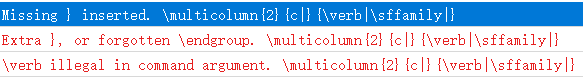# How to use \verb in the environment tabular?

I proposed to put a \verb|\sffamily| into a multicolumn command as the 3rd parameter, but LaTeX referred 3 errors. Here is my code.

\documentclass{article}
\usepackage{array}
\begin{document}
\begin{tabular}{|c|c|}\hline
\multicolumn{2}{c|}{\verb|\sffamily|}
\end{tabular}
\end{document}


And here is my errors## 4 Answers

The earlier answers cover the standard approaches to a case like this: use \texttt (either directly or through a new command), or somehow save the verbatim content in a normal context and then load it later.

Here is an alternate approach that uses fvextra. This requires v1.4, the newest version. fvextra now provides a \Verb command (note capitalization) that works inside other commands under most circumstances, with a few limitations such as no # or %. Details about limitations are in the documentation.

\documentclass{article}
\usepackage{array}
\usepackage{fvextra}
\begin{document}
\begin{tabular}{|c|c|}\hline
\multicolumn{2}{c|}{\Verb|\sffamily|}
\end{tabular}
\end{document}


fvextra now also provides a command \EscVerb for the cases when \Verb won't work inside another command. \EscVerb is similar to \Verb and the normal \verb, except that the backslash serves as an escape character (\\ becomes \, \# becomes #, etc.), and the argument must be delimited with a pair of curly braces.

\documentclass{article}
\usepackage{array}
\usepackage{fvextra}
\begin{document}
\begin{tabular}{|c|c|}\hline
\multicolumn{2}{c|}{\EscVerb{\\sffamily}}
\end{tabular}
\end{document}


Here is one way. Save the verbatim stuff in a verbbox before the tabular, and then invoke it from within the tabular.

\documentclass{article}
\usepackage{array,verbatimbox}
\begin{document}
\begin{myverbbox}{\sfverb}\sffamily\end{myverbbox}
\begin{tabular}{|c|c|}\hline
\multicolumn{2}{|c|}{\sfverb}\\
\hline
\end{tabular}
\end{document}Above, I use a named verbbox. If there is only one needed at a time, you can just use the default-named verbbox:

\documentclass{article}
\usepackage{array,verbatimbox}
\begin{document}
\begin{verbbox}\sffamily\end{verbbox}
\begin{tabular}{|c|c|}\hline
\multicolumn{2}{|c|}{\theverbbox}\\
\hline
\end{tabular}
\end{document}

• Yeah it's a nice solution, using box to fix this problem. – Syvshc Jan 31 at 14:11

You can't use \verb in the argument to another command, in this case \multicolumn.

For the specific problem, \verb is not necessary; I suggest to do

\newcommand{\cs}{\texttt{\symbol{\\}#1}}


and now

\multicolumn{2}{c|}{\cs{sffamily}}


will work.

• In other cases , we can use \cprotect to make \verb works. But this method seems not to work... – Syvshc Jan 31 at 14:09
• @Syvshc The package says “Include \verb anywhere!”, but it should say almost anywhere; the problem here is that \multicol must come first in a cell: either at the immediate beginning of a row or just after & (spaces are ignored). Using \cprotect\multicolumn defeats this. – egreg Jan 31 at 15:29
• I don't really understand what ''defeat'' here... – Syvshc Jan 31 at 15:32
• @Syvshc If \multicolumn is after \cprotect, it can't work. – egreg Jan 31 at 15:37

I would use verbdef to define a macro with the verbatim content outside the tabular and then use the macro inside it.

\documentclass{article}
\usepackage{array}
\usepackage{verbdef}
\begin{document}
\verbdef\myverbtext|\sffamily|
\begin{tabular}{|c|c|}\hline
\multicolumn{2}{c|}{\myverbtext}
\end{tabular}
\end{document}


The newverbs package also provides a \collectverb{<code>}<char><verbatim><char> macro which collects the verbatim for you and feeds it to a macro. However, \collectverb{\multicolumn{2}{c|}}|\sffamily| does not work here as \collectverb is not allowed between tabular rows (! Misplaced \omit.`).